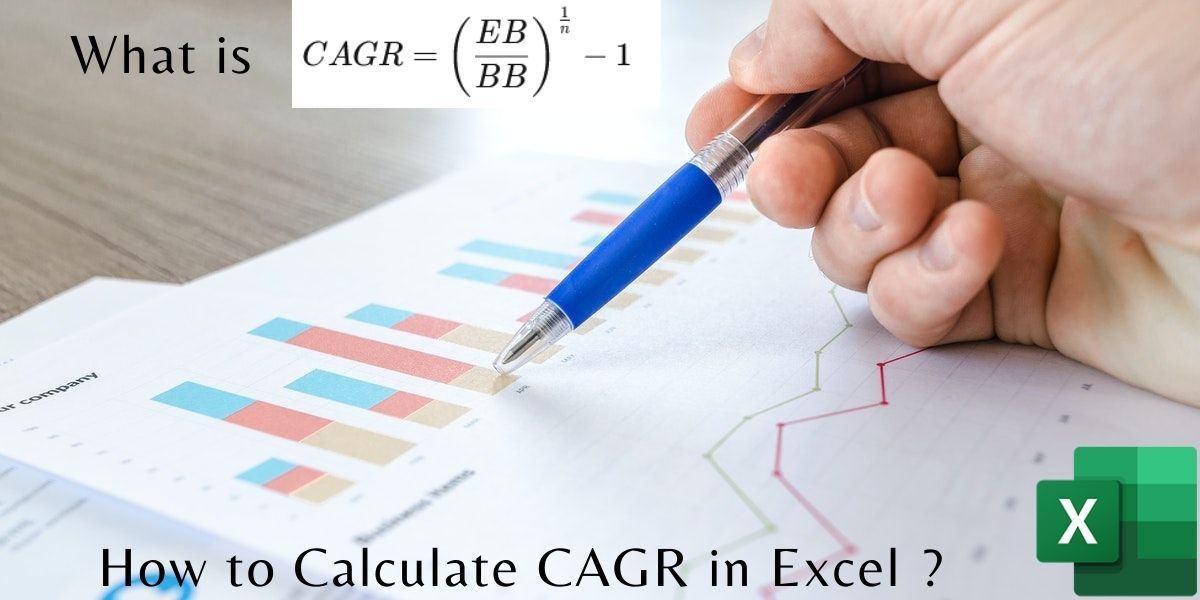# CAGR in Excel – How to Calculate CAGR in Excel?In this tutorial, we’ll learn how to calculate CAGR in Excel. CAGR or Compound Annual Growth Rate is the rate of measurement of the growth rate of an investment amount that is compounded annually.

Also read: How to Calculate Compound Interest in Excel?

The Formula to Calculate CAGR is:

This formula gives a CAGR value. But to express CAGR in percent(%), multiply the obtained CAGR value by 100.

## CAGR calculation Example

Let’s understand in detail using an example:

Let us assume, a person invested \$500 in 2018 for 3 years and his investment maturity is on 31st Dec, 2021 and his statement is as follows:

From the above statement, it is evident that from year-end 2018 to year-end 2019 the \$500 investment appreciated by 20% (from \$500 to \$600). Now from year-end 2019 to year-end 2020, following the Covid-19 impact the investment depreciated by 8.3% or -8.3% (from \$600 to \$550). Lastly, from year-end 2020 to year-end 2021 the investment grew by whopping 63.6% (from \$550 to \$900).

Substituting the above values, in the CAGR formula: CAGR = {[(900/500)^(1/3)] — 1} = 0.216. To express in general format x100% = 21.6%

Hence, at the end of 3 years of investment, the growth rate is 21.6% even after cycles of appreciation and depreciation the investment grew or appreciated at the rate of 21.6% annually.

Note: CAGR is only calculated for the fully completed years (12 months). Hence, n=3 in the example above.

## Calculate CAGR in Excel

Now let’s get right into calculating CAGR in Exel and learn from the methods outlined below.

### Method 1: Using RRI function

In this method, we’ll calculate CAGR using the RRI function in Excel. Let’s look into this now.

#### Step 1: Inserting the RRI function

• Select a cell where you want to display your result.
• Navigate to ‘Insert‘ and then click on ‘Function
• A pop up window will appear, select ‘RRI‘ function under ‘Finance‘ as shown in the below image.
`RRI (nper, pv, fv)  — Returns an equivalent interest rate for the growth of an investment`

Note:

1. Nper is the total number of periods. [ ‘n‘ in the CAGR formula]
2. Pv is the present value of the investment. [ ‘BB‘ Beginning Balance in the CAGR formula]
3. Fv is the future value of the investment. [ ‘EB‘ Ending Balance in the CAGR formula]

#### Step 2: Putting in Values in the function

• After selecting the function, choose the data as per the RRI formula inputs
• For nper you can either choose (row – 1) [-1 used as for completed years] or manually input the no. of years.

#### Step 3: Final Result

The Final Result of implementation of the RRI function to calculate CAGR (Compound Annual Growth Rate).

CAGR = 0.27038

To get the CAGR %, multiply by 100 in the RRI formula like [=RRI((A12-1), C2, C12) * 100] to get like

CAGR % = 27.038

### Method 2: CAGR in Excel Using a Custom Function

This method is fairly easy and direct to implement. In this method you are required to create the function yourself.

The formula to calculate CAGR is already mentioned here. You are required to implement it as is.

• Navigate to ‘Insert‘, then click on ‘Function‘.
• Choose custom formula option or Any other formula and clear the formatting of formula everything after the Equal (=) sign.